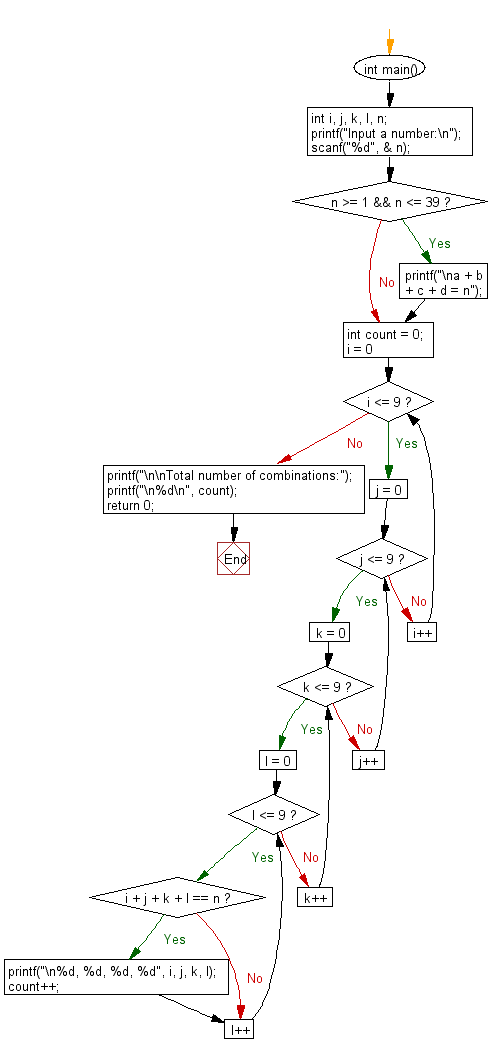﻿ C Program: Number of combinations of a, b, c and d

# C Exercises: Number of combinations of a, b, c and d (0 <= a, b, c, d <= 9) where (a + b + c + d) will be equal to n

## C Basic Declarations and Expressions: Exercise-135 with Solution

Write a C program that reads an integer n and finds the number of combinations of a, b, c and d (0 ≤ a, b, c, d ≤ 9) where (a + b + c + d) will be equal to n.

Input:
n (1 <= n <= 50)

Sample Solution:

C Code:

``````#include<stdio.h>

int main() {
int i, j, k, l, n;
printf("Input a number:\n");
scanf("%d", &n);

// Check if n is within the valid range (1 to 39)
if (n >= 1 && n <= 39) {
printf("\na + b + c + d = n");

// Initialize a counter to keep track of the valid combinations
int count = 0;

// Nested loops to iterate through all possible combinations of a, b, c, and d
for (i = 0; i <= 9; i++) {
for (j = 0; j <= 9; j++) {
for (k = 0; k <= 9; k++) {
for (l = 0; l <= 9; l++) {
if (i + j + k + l == n) {
// Print the combination and increment the counter
printf("\n%d, %d, %d, %d", i, j, k, l);
count++;
}
}
}
}
}

// Print the total number of valid combinations
printf("\n\nTotal number of combinations: %d\n", count);
}

return 0; // End of the program
}
``````

Sample Output:

```Input a number:
5

a + b + c + d = n
0, 0, 0, 5
0, 0, 1, 4
0, 0, 2, 3
0, 0, 3, 2
0, 0, 4, 1
0, 0, 5, 0
0, 1, 0, 4
0, 1, 1, 3
0, 1, 2, 2
0, 1, 3, 1
0, 1, 4, 0
0, 2, 0, 3
0, 2, 1, 2
0, 2, 2, 1
0, 2, 3, 0
0, 3, 0, 2
0, 3, 1, 1
0, 3, 2, 0
0, 4, 0, 1
0, 4, 1, 0
0, 5, 0, 0
1, 0, 0, 4
1, 0, 1, 3
1, 0, 2, 2
1, 0, 3, 1
1, 0, 4, 0
1, 1, 0, 3
1, 1, 1, 2
1, 1, 2, 1
1, 1, 3, 0
1, 2, 0, 2
1, 2, 1, 1
1, 2, 2, 0
1, 3, 0, 1
1, 3, 1, 0
1, 4, 0, 0
2, 0, 0, 3
2, 0, 1, 2
2, 0, 2, 1
2, 0, 3, 0
2, 1, 0, 2
2, 1, 1, 1
2, 1, 2, 0
2, 2, 0, 1
2, 2, 1, 0
2, 3, 0, 0
3, 0, 0, 2
3, 0, 1, 1
3, 0, 2, 0
3, 1, 0, 1
3, 1, 1, 0
3, 2, 0, 0
4, 0, 0, 1
4, 0, 1, 0
4, 1, 0, 0
5, 0, 0, 0

Total number of combinations:
56
```

Flowchart:C programming Code Editor:

What is the difficulty level of this exercise?

Test your Programming skills with w3resource's quiz.

﻿